# Groundwater Flow Calculation Model and Neural Network Prediction Based on Groundwater Modeling System

Groundwater Flow Calculation Model and Neural Network Prediction Based on Groundwater Modeling System
Institute of Urban and Rural Construction, Agricultural University of Hebei, Baoding 071001, China

Corresponding Author Email:
chengwuqun@126.com
Page:
461-470
|
DOI:
https://doi.org/10.18280/i2m.190607
1 July 2020
|
Accepted:
12 September 2020
|
Published:
29 December 2020
| Citation

OPEN ACCESS

Abstract:

Due to its merits of good water quality, pollution-free, and wide range, groundwater has become an important source for the water supply of industry, agriculture and urban domestic living in China. The change trends and laws of groundwater level and flow are important basis for the scientific planning and reasonable management of groundwater. In order to obtain a more accurate 3D groundwater flow model, first, this paper constructed a Groundwater Flow Calculation (GFC) model based on the Groundwater Modeling System (GMS), and analyzed the periodic groundwater recharge, the tridimensional flow space, the complex streamline combination, and the concurrent flow direction; then, the paper gave the preprocessing process of the calculated parameter data of groundwater flow, and built a grayscale-embedded BP neural network to predict the groundwater flow. At last, the paper verified the effectiveness of the model with experimental results. This study provided a reference for the flow prediction of other similar fields and the research on groundwater evaluation.

Keywords:

groundwater modeling system (GMS), groundwater flow prediction, BP neural network

1. Introduction

Groundwater is an important part of water resources in China. Due to its many merits of good water quality, pollution-free, wide range, stable water volume, and sustainable usage, it has now become one of the important sources for the water supply of industry, agriculture and urban domestic living [1, 2]. Although groundwater is buried in the ground, it has catchment areas and water flow systems just like rivers and lakes on the ground surface [3-7]. The change trends and laws of groundwater level and flow are important basis for the scientific planning and reasonable management of groundwater; and by controlling its trends, we can avoid problems such as the appearance of regional depression cones caused by over-exploitation [8-11]. Therefore, extracting the basic characteristics of the groundwater flows and comprehensively considering the tridimensional flow space, the complex streamline combination, and the concurrent flow direction are especially important for constructing high-precision and high-reliability groundwater model.

With the rapid development of computer technology, generalized numerical simulation models based on groundwater flow systems have been widely applied in foreign studies [12, 13]. In 1935, the appearance of the Theis formula had laid a foundation for the construction of unsteady flow models of groundwater flow systems . After the 1970s, Chinese scholars began to study groundwater flow systems. Hassane and Ackerer  built a model for the groundwater flow system in the North Plain based on the GIS groundwater simulation database, and gave the corresponding water resources evaluation, showing the advantages of the GIS groundwater simulation database in visualization and high-density data processing. In terms of the groundwater simulation methods, compared with the finite volume method and characteristic finite element method, the finite element method and the finite difference method are more widely applied due to their merits of simple, more reliable, and better local approaching effects, etc. [16-18]. The finite difference method which is capable of simulating the water volume of glacial water sediment aquifers first appeared in 1968 and the finite element analysis method which can simulate the sediment aquifers was proposed in 1972 for the first time . Bobet  constructed a groundwater flow system model based on multi-scale thinking and gave quantitative hydrogeological evaluation results; it analyzed and solved the problem of groundwater seepage. Matos and Alves  adopted the finite element method to simulate the heterogeneous groundwater system; it established a groundwater unsteady flow equation and obtained more accurate calculation results of groundwater flow. Stanko et al.  applied the irregular-grid finite difference method to solve the underground aquifer flow. Various water flow and solute transport simulation software has been gradually developed and applied since the 1980s. Farhadian et al.  applied Visual MODFLOW to water resources budget calculation and water volume management of the Balasu water source; combining with a 3D groundwater flow model, it analyzed the impact of groundwater exploitation volume on future water flow. GMS is a piece of software with graphical interface and comprehensive functions, it has the merits of fast speed, accurate calculation and seamless connection with GIS data. Pacheco  used GMS and GIS to simulate the groundwater flow in city suburbs to study the impact of agricultural activities on the regional groundwater; then, based on the quantified groundwater level recovery rate in the suburbs, it gave a comprehensive assessment of groundwater volume and quality, and proposed different water mining plans.

The complex structure of groundwater flow systems, plus the local rainfall and temperature meteorological conditions, has brought great difficulties for accurate and real-time groundwater flow simulation. From the perspective of system engineering, a neural network that can accurately fit the hidden laws of groundwater regime data is very suitable for groundwater flow system simulation, and water level and water flow prediction. In order to obtain a more accurate 3D groundwater flow model, this paper constructed a GMS-based GFC model and the corresponding neural network. The main contents of the paper are: Section 2 gave the groundwater flow measurement method based on GMS software; Section 3 analyzed the periodic groundwater recharge, the tridimensional flow space, the complex streamline combination, and the concurrent flow direction; it also gave the preprocessing process of calculated parameter data of groundwater flow; Section 4 constructed a grayscale-nested BP neural network to predict groundwater flow, and verified the effectiveness of the proposed model with experimental results.

2. Construction of the GMS-Based GFC Model

This paper drew on the conceptual modeling method of GMS software to model the groundwater flow system. First, according to hydrogeological maps and borehole data, a physical model of the study area had been built and converted into a grid model, then, generalized map data such as boundary conditions, hydrogeological parameters, and source sink term generated by the Map module were taken as the input of the model to generate the MODFLOW model and complete the calculation.

The simulation range of the groundwater flow system was centered on the study area of an unnatural hydrological boundary, and it expanded about 4-5km outward. The study area was about 4.7km and 5km from the left and right boundaries of the simulation range, and about 4.5km and 4.6km from the upper and lower boundaries; the simulation area was about 211.15km2. The grid model had 4 layers, including 100 rows and 100 columns. Figure 1 shows the simulation range of the groundwater flow system in the study area.

## 1.png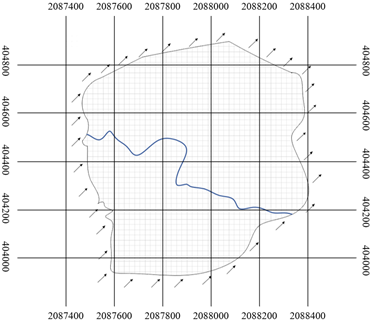Figure 1. Simulation range of the phreatic layer of the groundwater flow system in the study area

Table 1. Hydrogeological parameters of the study area

 Loose rock mass Permeability coefficient μA Px Py Pz Gravel 0.335 0.335 0.0335 0.00220 Coarse sand 0.0925 0.0925 0.00925 0.00120 Medium sand 0.00784 0.00784 0.000784 0.00040 Fine sand 0.00256 0.00256 0.00056 0.00021

The groundwater flow form can be equivalently regarded as a three-dimensional unsteady flow movement in which the flow direction and movement parameters would change with time and space in real time. Suppose the groundwater phreatic level is h; the aquifer level is TH; the permeability coefficients of each aquifer along the x, y, and z directions are Px, Py, and Pz; the water storage rate is WS; and the intensity of the source sink term is ηS. The 3D unsteady flow model of groundwater in the study area can be expressed by Formula 1:

\begin{align} & WS\frac{\partial TH}{\partial t}=\frac{\partial }{\partial x}\left( {{P}_{x}}\frac{\partial TH}{\partial x} \right)+\frac{\partial }{\partial y}\left( {{P}_{y}}\frac{\partial TH}{\partial y} \right) \\ & \text{ }+\frac{\partial }{\partial z}\left( {{P}_{z}}\frac{\partial TH}{\partial z} \right)+{{\eta }_{S}} \\\end{align}     (1)

Assume the water yield of the phreatic aquifer is μA, it can be calculated by the water level recovery method. Then there is:

\begin{align} & {{\mu }_{A}}\frac{\partial h}{\partial t}={{P}_{x}}{{\left( \frac{\partial h}{\partial x} \right)}^{2}}+{{P}_{y}}\left( \frac{\partial h}{\partial y} \right)+{{P}_{z}}\left( \frac{\partial h}{\partial z} \right) \\ & \text{ }-{{P}_{z}}{{\left( \frac{\partial h}{\partial z} \right)}^{2}}-{{P}_{z}}\frac{\partial h}{\partial z}+RE \\\end{align}      (2)

where, RE is the rainfall and evaporation of the phreatic aquifer. Table 1 shows the permeability coefficient and water yield of the study area. All the above-mentioned hydrogeological parameters were determined according to the collected borehole data and test data. Assume the length of the borehole filter is LF, DWL is the water level drop, the empirical formula for the aquifer permeability coefficient is:

$P=\frac{0.366IF}{{{L}_{F}}{{D}_{WL}}}\lg \frac{0.66{{L}_{F}}}{{{R}_{H}}}$     (3)

where, R and IF are respectively the borehole radius and water inflow. The water level recovery value R*WL after water pumping, and the remaining water level drop R'WL satisfy the relationship shown as Formula 4:

${{{R}'}_{WL}}={{R}_{S}}-R_{WL}^{*}$     (4)

where, RS is the water level drop by the end of water pumping. Assume the hydraulic conductivity of the aquifer is δ, R*WL can be written in the form of Formula 5 according to the principle of groundwater dynamics:

$R_{WL}^{*}=\frac{2.3IF}{4\pi \delta }\lg \frac{2.25t\delta }{{{R}_{H}}^{2}{{\mu }_{A}}}$     (5)

Order the moment when R*WL=0 be time t0, μA can be calculated by Formula 6:

$\mu =\frac{2.25T{{t}_{0}}}{{{r}^{2}}}$     (6)

The calculated parameters need to be substituted into the groundwater flow system model for verification and adjustment. The model boundary conditions characterizing the relationship between groundwater and its surrounding environment can be divided into three types: constant waterhead boundary, given flow boundary and mixed boundary. Figure 2 shows the boundary conditions of the study area after verification and adjustment. Suppose the initial groundwater level is h0; the aquifer boundary water level is TH'; B0, B1, and B2 are the groundwater free surface, waterhead first-class boundary, and flow second-class boundary, respectively. The fixed waterhead boundary can be expressed by Formula 7:

$TH\left( x,y,z,t \right)\left| _{{{B}_{1}}}=T{H}'\left( x,y,z,t \right) \right.$     (7)

## 2.png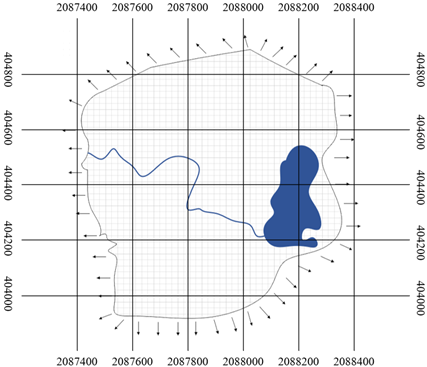Figure 2. Boundary conditions of the artesian aquifer of the groundwater flow system in the study area (including catchment area)

The given flow boundary can be expressed by Formula 8:

${{\left. \sigma \frac{\partial TH}{\partial d} \right|}_{{{B}_{2}}}}={{f}_{p}}\left( x,y,z,t \right)$      (8)

where, fp and d are the flow per unit area and the normal direction on B2, respectively. Assume a and b are known functions, and the mixed boundary can be expressed by Formula 9:

$\frac{\partial TH}{\partial d}+aTH=b$     (9)

Based on the initial water-level flow field, the model boundaries were subject to the constant flow boundary generalization processing. The left and right boundaries parallel to the water level contour were generalized as flow recharge and discharge boundaries, and the upper and lower boundaries perpendicular to the contour were generalized as the zero-flow impervious boundaries. Drawing on Darcy's formula, lateral recharge and discharge flow SD could be calculated as shown in Formula 10:

$SD={{P}_{av}}\cdot {{T}_{av}}\cdot H{{G}_{av}}\cdot {{L}_{S}}$      (10)

where, Pav is the average value of permeability coefficient; Tav and HGav are the average values of section thickness and hydraulic gradient, respectively; LS is the section length. The calculated SD can be imported into the boundary of the constructed groundwater flow system model. Table 2 gives the groundwater recharge and discharge parameters in the study area.

Table 2. Table of groundwater recharge and discharge parameters

 Aquifer Boundary Pav LS Tav HGav SD Phreatic layer Lateral recharge 3.75 5.8 27 0.045 26.42 Lateral discharge 3.75 5.2 24 0.037 17.31 Artesian layer Lateral recharge 0.14 5.8 43 0.046 1.606 Lateral discharge 0.14 5.2 33 0.034 0.817

The groundwater recharge of the model was mainly supplied by rainfall, the stop time of rainfall recharge of the study area and the recharge speed were also imported into the model. The recharge speed can be calculated by Formula 11:

${{V}_{RR}}=\beta \cdot R{{F}_{D}}$     (11)

where, β is the rainfall infiltration coefficient determined by the surface conditions of the study area, and RFD is the average daily rainfall of the study area. Figure 3 shows the monthly rainfall in the study area in 2019.

## 3.pngFigure 3. Monthly rainfall in the study area in 2019

Table 3. Monthly groundwater evaporation

 Month Evaporation intensity Month Evaporation intensity January 0.00254 February 0.00110 March 0.00089 April 0.00154 May 0.00189 June 0.0247 July 0.00452 August 0.00415 September 0.00578 October 0.00359 November 0.00268 December 0.00209

The groundwater evaporation of the model was mainly characterized by the groundwater free surface evaporation intensity obtained by multiplying the evaporation coefficient and the average groundwater evaporation. Table 3 summarizes the monthly groundwater evaporation intensity in the study area in 2019.

3. Characteristic Analysis and Preprocessing of Calculated Parameter Data of Groundwater Flow

3.1 Characteristics analysis of parameter data

Because some variation characteristics of groundwater flow have a great influence on the calculation, it is necessary to analyze the periodic groundwater recharge, the tridimensional flow space, the complex streamline combination, and the concurrent flow direction of the groundwater recharge.

Since groundwater recharge is mainly supplied by rainfall, the periodicity of groundwater recharge needs to be analyzed according to the similarity and periodic characteristics of the rainfall data in the study area. The monthly increase or decrease of groundwater recharge was basically consistent with the rainfall amount, and the recharge peaks and troughs had certain regularity. Compared with the recharge amount from November to March of the following year, the rainfall recharge of the rain season from July to September can still maintain the regular peak and trough changes, and the total recharge amount showed a significant increase. The peak and trough water flow changes also raised higher requirements for the calculation performance of the model.

In a groundwater flow system, the flow space at any position is three-dimensional, and the cross-correlation of the flow space is equal to the value of the spatial distance function of the flow space. The spatial cross-correlation between two branch sections in a same flow path will increase with the increase in groundwater recharge. The structure of the water flow system is complex, and the characteristics of the water flow are particularly obvious at the water flow confluence. Assume the water flow in the directions of A, B, and C within the time period [(i-1)t,it] is a(i), b(i), and c(i), respectively; D(t) is the total water flow including a(i), b(i), and c(i); in terms of space, the groundwater flow at the confluence is related to the water flow in a(i), b(i), c(i) and other directions; this has also verified the complexity of the streamline combination of the groundwater flow system.

The groundwater flow system has the characteristics of concurrent flow direction and time correlation. The time series of groundwater flow has the fractal features that are positively correlated with the future and historical change trends. It shows certain regularity within a same time period, and the differences between different time periods are quite obvious. To predict the groundwater flow D(i+1) within the next time period [it,(i+1)t] by analyzing the time characteristics of the groundwater flow parameters in the study area, it is necessary to comprehensively consider the water flow in the first j time periods of the water flow branches at the confluence a(i),b(i),c(i),…,a(i+1-j),b(i+1-j),c(i+1-j), and the water flow D(i+1), D(i),…,D(i+1-j) in the first j time periods related to D(i+1).

3.2 Parameter data preprocessing

## 4.png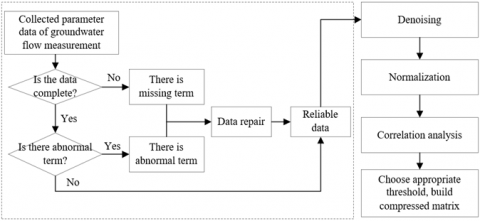Figure 4. Flow of parameter data preprocessing

Figure 4 shows the preprocessing flow of measured groundwater flow parameters. The details are as follows:

(1) Repair of abnormal parameter data

The abnormal groundwater flow parameter data was mainly divided into error data and missing data. Data with obvious errors can be directly eliminated, and missing data can be repaired by the historical trend method shown as Formula 12:

$D\left( t \right)=\lambda D\left( t \right)+\left( 1-\lambda \right)D\left( t-1 \right)$     (12)

According to the formula, the missing data was filled by the weighted sum of the water flow D(t-1) at time t-1 and the water flow D(t) at time t, where λ is the smoothing coefficient.

(2) Normalization of parameter data

When constructing the groundwater flow prediction model, in order to avoid the saturation of neural network neurons, it is necessary to normalize the measured parameter data. Since groundwater flows are all positive values, this paper limited the numerical value characterizing the magnitude of water flow to the interval [0, 1]. For the groundwater flow sequence f1, f2, ..., fn, the Min-Max method was adopted to perform the normalization shown as Formula 13:

${{{f}'}_{i}}=\frac{{{f}_{i}}-\underset{1\le j\le n}{\mathop{\min }}\,\left\{ {{f}_{j}} \right\}}{\underset{1\le j\le n}{\mathop{\max }}\,\left\{ {{f}_{j}} \right\}-\underset{1\le j\le n}{\mathop{\min }}\,\left\{ {{f}_{j}} \right\}}$     (13)

(3) Construction of the compressed matrix of parameter data

## 5.png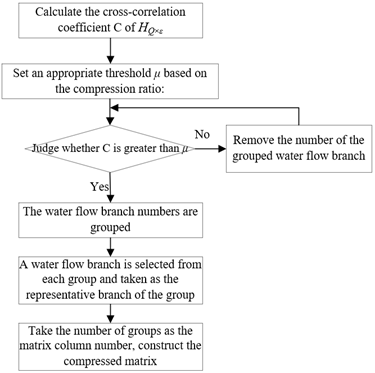Figure 5. Construction process of compressed matrix of measured parameters

Figure 5 shows the construction process of the compressed matrix of the measured parameter data. First, the correlation coefficient C between two parameters is defined as:

$C=\frac{\sum\limits_{i=1}^{n}{\left( {{a}_{i}}-\bar{a} \right)}\left( {{b}_{i}}-\bar{b} \right)}{\sqrt{\sum\limits_{i=1}^{n}{{{\left( {{a}_{i}}-\bar{a} \right)}^{2}}\sum\limits_{i=1}^{n}{{{\left( {{b}_{i}}-\bar{b} \right)}^{2}}}}}}$     (14)

where, ai and bi are respectively the calculated value and the detected value of the measured parameters; $\bar{a}$ and $\bar{b}$ are the average values obtained by multiple calculations or detections.

Construct a network graph ND=(Nnode,Abranch) for the groundwater flow system in the research area shown in Figure 2, where Nnode is the number of nodes in the groundwater flow system; Abranch is the collection of all water flow branches in the system. Correspondence can be established between the parameter data of all water flow branches in the groundwater flow system and the mathematical formulas. Suppose there are Q flow branches in the groundwater flow system, represented by Abranch={Ai,i=12,…,Q}, ε is the time lag of history water flow data. Then any branch Ai contains a continuous time series representing the water flow of the branch in the time period (t-ε+1,t), denoted as Di={D(Ai,t-ε+1), D(Ai,t-ε+2),…,D(Ai,t)}. The water flow data of the entire groundwater flow system constitutes the two-dimensional space-time matrix shown in Formula 15:

\begin{align} & {{H}_{Q\times \varepsilon }}=\left[ \begin{matrix} {{H}_{1}} \\ {{H}_{2}} \\ ... \\ {{H}_{Q}} \\\end{matrix} \right] \\ & =\left[ \begin{matrix} D\left( {{A}_{1}},t-\varepsilon +1 \right) & D\left( {{A}_{1}},t-\varepsilon +2 \right) & ... & D\left( {{A}_{1}},t \right) \\ D\left( {{A}_{2}},t-\varepsilon +1 \right) & D\left( {{A}_{2}},t-\varepsilon +2 \right) & ... & D\left( {{A}_{2}},t \right) \\ ... & ... & ... & ... \\ D\left( {{A}_{Q}},t-\varepsilon +1 \right) & D\left( {{A}_{Q}},t-\varepsilon +2 \right) & ... & D\left( {{A}_{Q}},t \right) \\\end{matrix} \right] \\\end{align}     (15)

The correlation coefficient C(k,l) of any two flow branches in the groundwater flow system can be calculated by Formula 16:

\begin{align} & C\left( k,l \right) \\ & =\frac{\sum\limits_{s=t-\varepsilon +1}^{t}{\left( D\left( {{A}_{k}},{{t}_{s}} \right)-\bar{D}\left( {{A}_{k}} \right) \right)\left( D\left( {{A}_{l}},{{t}_{s}} \right)-\bar{D}\left( {{A}_{l}} \right) \right)}}{\sqrt{\sum\limits_{s=t-\varepsilon +1}^{t}{{{\left( D\left( {{A}_{k}},{{t}_{s}} \right)-\bar{D}\left( {{A}_{k}} \right) \right)}^{2}}}}\sqrt{\sum\limits_{s=t-\varepsilon +1}^{t}{{{\left( D\left( {{A}_{l}},{{t}_{s}} \right)-\bar{D}\left( {{A}_{l}} \right) \right)}^{2}}}}} \\\end{align}     (16)

where, $\bar{D}\left(A_{k}\right)$ and $\bar{D}\left(A_{l}\right)$  represent the cross-correlation coefficients of the water flow branches Ak and Al, and the size of C(k,l) represents the degree of correlation between the two branches. By comparing the cross-correlation coefficient C of HQ×ε and the size of preset threshold μ, the numbered branches can be grouped, and a representative branch can be selected from each group. With the number of groups as the matrix column number, the compressed matrix of the water flow system was constructed. Table 4 shows the correlation between threshold μ and compression ratio CR and the running time of the model. According to the table, threshold μ determines the number of water flow branches in the compressed matrix, and the setting of its size will directly affect the accuracy of groundwater flow calculation. The compression ratio in Formula 17 can be used to set an appropriate value for threshold μ:

$CR=\frac{Q}{{{N}_{group}}}\times 100%$     (17)

Table 4. The correlation between threshold μ and compression ratio CR and model running time

 μ 0.86 0.88 0.90 0.92 0.94 0.96 0.98 1 CR 32.3% 28.4% 17.7% 12.6% 9.74% 7.26% 5.7% 1 Running time/Second 24.75 33.21 45.34 56.78 60.24 71.85 78.91 89.7
4. Construction of Grayscale-Embedded BP Neural Network

The actually measured parameter data of groundwater flow has the characteristics of the gray system, that is, it has a certain degree of randomness and periodicity. This paper selected the GM (1, 1) model as the embedded model for the BP neural network, the GM (1, 1) model is suitable for original parameter data sequence with positive values and low discrete levels, and it is suitable for short-term groundwater flow prediction. During the prediction process, the groundwater flow parameter data was added and updated in real time so as to ensure the validity and accuracy of the prediction results. The model can be solved through the following steps:

(1) A less-correlated non-negative original groundwater flow parameter time series fp0 is established as shown in Formula 18:

$f_{p}^{0}=\left\{ f_{1}^{0},f_{2}^{0},...,f_{n}^{0} \right\}$     (18)

The first-order accumulation result of fp0 is:

$f_{p}^{1}=\sum\limits_{i=1}^{p}{f_{i}^{0}}$     (19)

which can be re-written in the data column form as follows:

$f_{{}}^{1}=\left\{ f_{1}^{1},f_{2}^{1},...,f_{n}^{1} \right\}$     (20)

(2) Suppose the development coefficient is τ, and the gray action is φ, a first-order linear differential equation can be constructed as shown in Formula 21:

$\frac{\text{d}f_{{}}^{1}}{dt}+\tau f_{{}}^{1}=\phi$     (21)

In the formula, τ reflects the development trend of the time series f0 and f1 of groundwater flow parameters, its value range is -2~2. As long as the gray parameter matrix Φ=(τ, φ)T of the equation is determined, f1 can be solved and its future value could be predicted.

(3) Construct the constant term vector of the parameters as shown in Formula 22:

CT=\left( \begin{align} & f_{2}^{0} \\ & f_{3}^{0} \\ & \text{ }\ldots \\ & f_{m}^{0} \\\end{align} \right)     (22)

Calculate the average value of the time series elements to construct the matrix shown in Formula 23:

MC=\left[ \begin{align} & -\frac{\left( f_{1}^{1}+f_{2}^{1} \right)}{2} 1 \\ & -\frac{\left( f_{2}^{1}+f_{3}^{1} \right)}{2} 1 \\ & ... \\ & -\frac{\left( f_{m-1}^{1}+f_{m}^{1} \right)}{2} \text{ }1 \\\end{align} \right]     (23)

The number of established m-1 differential equations is greater than 2, and the approximate solution of Φ is solved using the least square method shown as Formula 24:

$\Phi ={{\left( M{{C}^{T}}MC \right)}^{-1}}M{{C}^{T}}CT$     (24)

(4) Under the initial condition of f10=f11=11, the solution of the differential equation is the response equation of the whitening differential equation of the GM (1,1) model. Substitute Φ into Formula 22 and solve it, then the prediction model of f1 is obtained as:

${f}_{p+1}^{1}=\left( f_{1}^{0}{{x}^{\left( 0 \right)}}\left( 1 \right)-\frac{\phi }{\tau } \right){{e}^{-\tau p}}+\frac{\phi }{\tau }$     (25)

(5) The approximate reduction of the original measured parameter sequence p+11 can be completed by Formula 26:

${f}_{p+1}^{0}={f}_{p+1}^{1}\text{-}{f}_{p}^{1}$     (26)

(6) Perform the test on the constructed GM (1,1) model, as shown in Formula 27:

$\left\{ \begin{matrix} RES_{p}^{0}=f_{p}^{0}-{f}_{p}^{0} \\ RE_{p}^{0}=RES_{p}^{0}/{f}_{p}^{0} \\\end{matrix} \right.$     (27)

where, RESp0 and REp0 are respectively the residual and relative error of the model.

(7) The generation of future groundwater flow prediction data was mainly completed by the basic formulas of the GM (1,1) model shown as Formulas 25 and 26, and the prediction result can be expressed by Formula 28:

${f}_{{}}^{0}=\left\{{f}_{1}^{0},{f}_{2}^{0},...,{f}_{m}^{0},{f}_{m+1}^{0},...,{f}_{m+n}^{0} \right\}$     (28)

In the above formula, the first m terms are the fitted values of the time series of the original measured parameter data of the groundwater flow, and the last n terms are the prediction values of the short-term groundwater flow.

For the constructed grayscale-embedded BP neural network, the input layer neuron nodes only considered some variation characteristics of groundwater flow contained in the original measured parameter data sequence, and did not consider the impact of external environment on the water flow. In the actual application of groundwater flow prediction in the study area, it is found that the prediction results of the constructed model had not reached the expected error level. For this reason, an anti-interference correction coefficient was proposed for the model, and the verification of the value of the correction coefficient can be completed based on the Markov model as shown in Formula 29:

${{\sigma }_{t+1}}={{\sigma }_{0}}{{\left[ {{\sigma }^{1}} \right]}^{t+1}}$     (29)

where, σt+1 is the probability distribution of the model's water flow prediction results at time t+1, and σ0 is the unconditional probability distribution of the prediction results at the initial time moment. The one-step transition probability matrix σ1 of the Markov model can be calculated by Formula 30:

${{\sigma }^{1}}=\left[ \begin{matrix} {{\sigma }_{11}} & {{\sigma }_{12}} & ... & {{\sigma }_{1n}} \\ {{\sigma }_{21}} & {{\sigma }_{21}} & ... & {{\sigma }_{2n}} \\ ... & ... & ... & ... \\ {{\sigma }_{n1}} & {{\sigma }_{n1}} & ... & {{\sigma }_{nn}} \\\end{matrix} \right]$     (30)

The elements in the matrix represent the probability of changes in the prediction values of water flow in a unit time period.

5. Experimental Results and Analysis

When stimulating the groundwater flow system using the constructed model, the core reference standard is the reasonably estimated initial water levels of the phreatic layer and the artesian layer. Combining with the drilling data of January 2019 and the dynamic data of groundwater flow parameter measurement, the initial water level of the study area can be obtained and imported into the constructed model for groundwater flow calculation and prediction. Figures 6 and 7 respectively show the initial water levels of the phreatic layer and artesian layer of the groundwater system in the study area.

## 6.png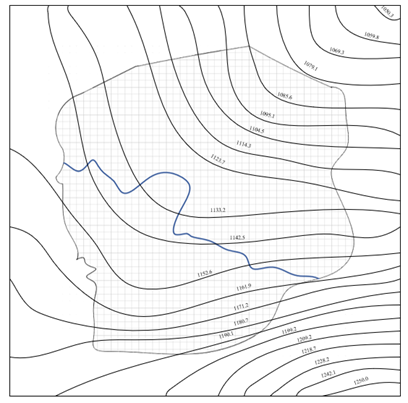Figure 6. Initial water level of phreatic layer in the study area

## 7.png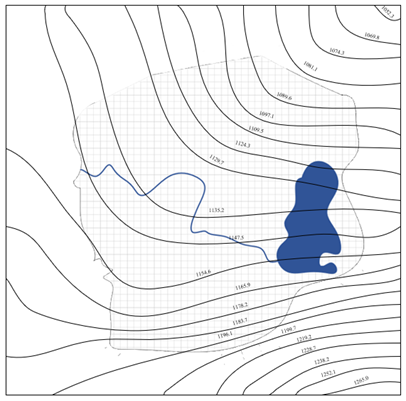Figure 7. Initial water level of artesian layer in the study area (including catchment area)

In order to verify the accuracy of the proposed neural network for the prediction of groundwater flow in the study area, the water flow results of a same groundwater branch calculated by GMS software were respectively trained and simulated by the traditional BP neural network and the proposed neural network. 45 groups of measured parameter data of groundwater flow were taken as training samples, and the other 15 groups were taken as test samples. Table 5 shows the measurement and prediction data of 8 sample points. Figure 8 intuitively fits the predicted data of the two models and compares them with the measured values. In the figure, the ordinate is the groundwater flow value, the abscissa is the number of sample points. According to the figure and table, compared with the traditional BP neural network, the proposed neural network had smaller relative error and higher accuracy.

Table 5. Some measured values and predicted values under different model conditions

 No. Measured value Traditional BP neural network Proposed neural network Predicted value Relative error Predicted value Relative error 1 26.45 24.66 -6.76% 27.19 2.79% 2 32.71 35.27 7.82% 31.54 -3.57% 3 44.97 46.98 4.46% 45.21 0.53% 4 59.80 60.67 1.45% 58.97 -1.38% 5 67.94 74.98 10.36% 66.22 -2.53% 6 72.56 79.29 9.27% 74.32 2.42% 7 88.67 92.94 4.81% 88.10 -0.64% 8 95.98 98.09 2.19% 96.89 0.94% MSE 5.74*10-3 3.25*10-3

## 8.png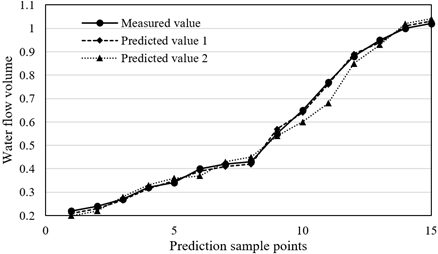Figure 8. Comparison results of measured values and predicted values of two models

Figure 9 shows the groundwater flow prediction results of a same sample point in different time periods. It can be seen from the figure that the proposed model had a good fitting effect on the groundwater flow prediction values in different time periods, and the accuracy can reach the prediction requirement. The proposed model was highly reliable and can be applied to the study area.

## 91.png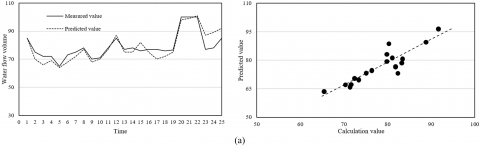## 92.png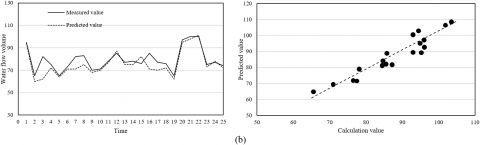## 93.png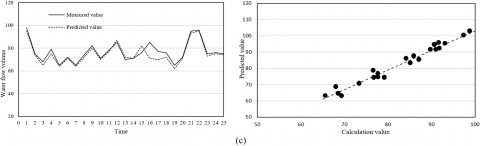## 94.png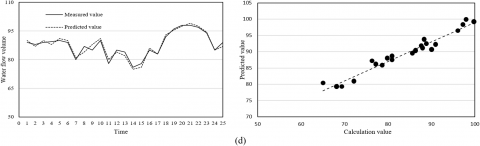Figure 9. Prediction results of groundwater flow different time periods

6. Conclusion

This paper constructed a GMS-based GFC model and a grayscale-embedded BP neural network. First, the GFC model was constructed based on GMS, and the characteristics of parameter data were analyzed from four aspects: the periodic groundwater recharge, the tridimensional flow space, the complex streamline combination, and the concurrent flow direction; and the preprocessing process of the measured parameter data of groundwater flow was given. Then, the paper constructed a neural network model to predict groundwater flow, and experiment gave the initial water levels of the phreatic layer and the artesian layer of the groundwater flow system in the study area. Through comparative experiments, the paper verified that the proposed neural network achieved smaller relative error and higher accuracy in water flow prediction due to the embedded GM (1,1) gray scale model. It had better groundwater flow prediction effects in different time periods and it had higher reliability in applications.

Acknowledgment

This paper was supported by the Open Fund project of State Key Laboratory of Hydroscience and Engineering of Tsinghua University (Grant No.: sklhse-2020-A-01).

References

 Al-Muqdadi, S.W., Abo, R., Khattab, M.O., Abdulhussein, F.M. (2020). Groundwater flow-modeling and sensitivity analysis in a hyper arid region. Water, 12(8): 2131. https://doi.org/10.3390/w12082131

 Ji, X., Luo, M., Wang, X.S. (2020). Accelerating streamline tracking in groundwater flow modeling on GPUs. Groundwater, 58(4): 638-644. https://doi.org/10.1111/gwat.12959

 Kamath, R.P., Unnikrishnan, N. (2020). Investigations on the impact of sub-structures on groundwater flow. In Construction in Geotechnical Engineering, pp. 557-564. https://doi.org/10.1007/978-981-15-6090-3_40

 Banks, A.T., Phetheet, J., Hill, M.C. (2020). An interactive computer module for understanding groundwater flow and transport. Groundwater, 6(58): 868-871. https://doi.org/10.1111/gwat.13040

 Morgan, F.D., Al Nasser, S., Jerry, R., Verneuil, A. (2019). Investigations into groundwater flow towards a spring in the Saphire Area, Soufriere, St Lucia, West Indies. SEG Technical Program Expanded Abstracts, 2772-2776. https://doi.org/10.1190/segam2019-3215507.1

 Langford, J.E., Schincariol, R.A., Nagare, R.M., Quinton, W.L., Mohammed, A.A. (2020). Transient and transition factors in modeling permafrost thaw and groundwater flow. Groundwater, 58(2): 258-268. https://doi.org/10.1111/gwat.12903

 Warix, S.R., Rademacher, L.K., Meyers, Z.P., Frisbee, M.D. (2020). Groundwater geochemistry and flow in the Spring Mountains, NV: Implications for the Death Valley regional flow system. Journal of Hydrology, 580: 124313. https://doi.org/10.1016/j.jhydrol.2019.124313

 Bailey, R.T., Park, S., Bieger, K., Arnold, J.G., Allen, P.M. (2020). Enhancing SWAT+ simulation of groundwater flow and groundwater-surface water interactions using MODFLOW routines. Environmental Modelling & Software, 126: 104660. https://doi.org/10.1016/j.envsoft.2020.104660

 Litvinenko, A., Logashenko, D., Tempone, R., Wittum, G., Keyes, D. (2020). Solution of the 3D density-driven groundwater flow problem with uncertain porosity and permeability. GEM-International Journal on Geomathematics, 11(1): 1-29. https://doi.org/10.1007/s13137-020-0147-1

 Barna, J.M., Fryar, A.E., Cao, L., Currens, B.J., Peng, T., Zhu, C. (2020). Variability in groundwater flow and chemistry in the Houzhai Karst Basin, Guizhou Province, China. Environmental & Engineering Geoscience, 26(3): 273-289. https://doi.org/10.2113/EEG-2306

 Bense, V.F., Kurylyk, B.L., de Bruin, J.G.H., Visser, P. (2020). Repeated subsurface thermal profiling to reveal temporal variability in deep groundwater flow conditions. Water Resources Research, 56(6): e2019WR026913. https://doi.org/10.1029/2019WR026913

 Kavvas, M.L., Tu, T., Ercan, A., Polsinelli, J. (2020). Fractional governing equations of transient groundwater flow in unconfined aquifers with multi-fractional dimensions in fractional time. Earth System Dynamics, 11(1): 1-12. https://doi.org/10.5194/esd-11-1-2020

 Park, Y.J., Hwang, H.T., Suzuki, S., Saegusa, H., Nojiri, K., Tanaka, T., Bruines, P., Abumic, K., Moritad, Y., Illmane, W.A. (2020). Improving precision in regional scale numerical simulations of groundwater flow into underground openings. Engineering Geology, 274: 105727. https://doi.org/10.1016/j.enggeo.2020.105727

 Dong, G., Tian, J., Zhan, H., Liu, R. (2017). Groundwater flow determination using an interval parameter perturbation method. Water, 9(12): 978. https://doi.org/10.3390/w9120978

 Hassane, M.M.F., Ackerer, P. (2017). Groundwater flow parameter estimation using refinement and coarsening indicators for adaptive downscaling parameterization. Advances in Water Resources, 100: 139-152. https://doi.org/10.1016/j.advwatres.2016.12.013

 Erdal, D., Cirpka, O.A. (2017). Preconditioning an ensemble Kalman filter for groundwater flow using environmental-tracer observations. Journal of Hydrology, 545: 42-54. https://doi.org/10.1016/j.jhydrol.2016.11.064

 Comte, J.C., Wilson, C., Ofterdinger, U., González-Quirós, A. (2017). Effect of volcanic dykes on coastal groundwater flow and saltwater intrusion: A field-scale multiphysics approach and parameter evaluation. Water Resources Research, 53(3): 2171-2198. https://doi.org/10.1002/2016WR019480

 Atangana, A.,Ünlü, C. (2016). New groundwater flow equation with its exact solution. Scientia Iranica, 23(4): 1837-1843. https://doi.org/10.24200/SCI.2016.3930

 Salama, S.M., Bekhit, H.M., Hassan, A.E. (2016). Solving variable density groundwater flow using random walk particle tracking method. Journal of Engineering and Applied Science, 63(2): 79-99.

 Bobet, A. (2016). Deep tunnel in transversely anisotropic rock with groundwater flow. Rock Mechanics and Rock Engineering, 49(12): 4817-4832. https://doi.org/10.1007/s00603-016-1118-6

 Matos, A.P., Alves, C. (2016). Multivariate statistical analysis of hydrogeochemical data towards understanding groundwater flow systems in granites. Quarterly Journal of Engineering Geology and Hydrogeology, 49(2): 132-137. https://doi.org/10.1144/qjegh2016-006

 Stanko, Z.P., Boyce, S.E., Yeh, W.W.G. (2016). Nonlinear model reduction of unconfined groundwater flow using POD and DEIM. Advances in Water Resources, 97: 130-143. https://doi.org/10.1016/j.advwatres.2016.09.005

 Farhadian, H., Katibeh, H., Huggenberger, P. (2016). Empirical model for estimating groundwater flow into tunnel in discontinuous rock masses. Environmental Earth Sciences, 75(6): 471. https://doi.org/10.1007/s12665-016-5332-z

 Pacheco, F.A. (2015). Regional groundwater flow in hard rocks. Science of the Total Environment, 506: 182-195. https://doi.org/10.1016/j.scitotenv.2014.11.008